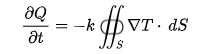# Laws Of Physics

The laws of physics are by their very nature facts that have been deduced and derived on the basis of empirical observations. Quite simply, the world around us works in a certain way and the laws of physics are a way of classifying that “work”.

Physical laws are nothing but conclusions drawn on the basis of years (or so) of scientific observations and experiments repeated over and over under different conditions to reach universally accepted conclusions. They are continuously validated by the scientific community over time. See the list of basic laws of physics below.5 Important Laws of Physics

• Lambert’s Cosine Law

We have studied various concepts in optics in our previous sessions. We came across different laws like Snell’s law, the laws of reflection and many others. In this article we will learn Lambert’s law of cosines and the derivative of luminous flux.

## Lambert’s Cosine Law Explanation

Lambert’s cosine law states that the surface radiation intensity and the ideal reflection cosine of the angle θ between the direction of the incident light and the surface normal are directly proportional. This law is named after Johann Heinrich Lambert, who is studied in optics and is also known as Lambert’s emission law or Cosine emission law.

# Kelvin-Planck Statement

• ##### What is the Kelvin Planck Statement?

This is a special case of the second law of thermodynamics. He states that “it is impossible to build an apparatus which works on a cycle and produces no other effect than the transmission of heat from a single body to produce work”. This means that it is impossible to build an engine whose sole purpose is to convert heat from a high temperature source/tank into an equal amount of work.

We know that heat (Q) and work (W) are the two forms of energy. Both follow the SI Joules unit and both are interconvertible. It allows work to be converted into heat and vice versa. Here, work can be completely converted into heat, but heat cannot be completely converted into work. Therefore, work is called high-quality energy, and heat is called low-quality energy.

The Kelvin-Planck statement consists of two different statements from Lord Kelvin and Planck. They are known as the Kelvin statement and the Planck statement.

Kelvin’s statement stated that it is impossible to obtain the mechanical effect of any substance by cooling it below the maximum cooling temperature of the surrounding objects.

Planck’s theorem states that the sum of entropies of the reversible system remains constant.

By understanding these two statements, the Kelvin-Planck statement was derived.

The Kelvin-Planck statement, also known as the second law of thermodynamics for the heat engine, states that it is impossible to design a device that runs on a cycle and produces no effect other than heat transfer. labor production.

Clausius Statement

• ##### Who was Rudolf Clausius?

Rudolf Julius Emanuel Clausius, a German mathematician and physicist, is one of the central founders of the science of thermodynamics. There are some important concepts introduced by Rudolf Clausius, one of which is the theory of heat, which is a reformulation of the Carnot cycle. In 1850 he published an article in “The Motive Force of Heat”. In 1865 he introduced entropy and the virial theorem in 1870.

# Fourier’s Law

We know that heat conduction occurs when matter molecules vibrate. Thermal energy is transferred from an area of higher temperature to an area of lower temperature. This process follows Fourier’s law. We understand Fourier’s law through the following article. Fourier’s law is also called the law of heat conduction equations or the law of thermal conductivity.

• ##### What Is Fourier’s Law?

Fourier’s law states that the negative temperature gradient and the rate of heat transfer are proportional to the area of the right angle of this gradient through which the heat flows. Fourier’s law is another name for the law of heat conduction.

Newton’s law of cooling and Ohm’s law are a discrete electrical analog to Fourier’s law.

## Differential Form Of Fourier’s Law

Fourier’s law differential form is as follows:

 q=−k▽T

Where,

• q is the local heat flux density in W.m2
• k is the conductivity of the material in W.m-1.K-1
• ▽T is the temperature gradient in K.m-1

In one-dimensional form:

 qx=−kdTdx

Integral formWhere,

• ∂Q∂t

is the amount of heat transferred per unit time

• dS is the surface area element

When the same equation is given in the differential form, which is the basis of heat equation derivation:

 Q△t=−kA(△T△x)

Where,

• A is the area of the cross-sectional surface
• ΔT is the temperature difference between the endpoints
• Δx is the distance between two ends

# Lagrangian Point

In astronomy, the Lagrangian point plays a fundamental role. It was important to know the points where the force exerted between two objects is equal. The object placed on the Lagrange point experiences the neutral force.

• ##### What is a Lagrangian Point?

A Lagrangian point is defined as the point near two large orbiting bodies such that the smaller object maintains its position relative to the large orbiting bodies. Lagrange points are also known as L points or Lagrange points or library points.

There are five Lagrangian points L1 to L5 for a given combination of two large orbital bodies. The first three Lagrangian points L1, L2 and L3 were discovered by Leonhard Euler and L4 and L5 were discovered by Joseph-Louis.

Of the five Lagrange points, three are unstable and two are stable. L1, L2 and L3 are unstable Lagrange points and lie along the line connecting the two large masses. L4 and L5 are stable Lagrangian points and form vertices of two equilateral triangles that have large masses at their vertices. In the Earth system, the Lagrangian first (L1) and second (L2) points are 1,500,000 km (900,000 mi) from Earth toward and away from the Sun. The Lagrangian of the Sun-Earth system hosts satellites.